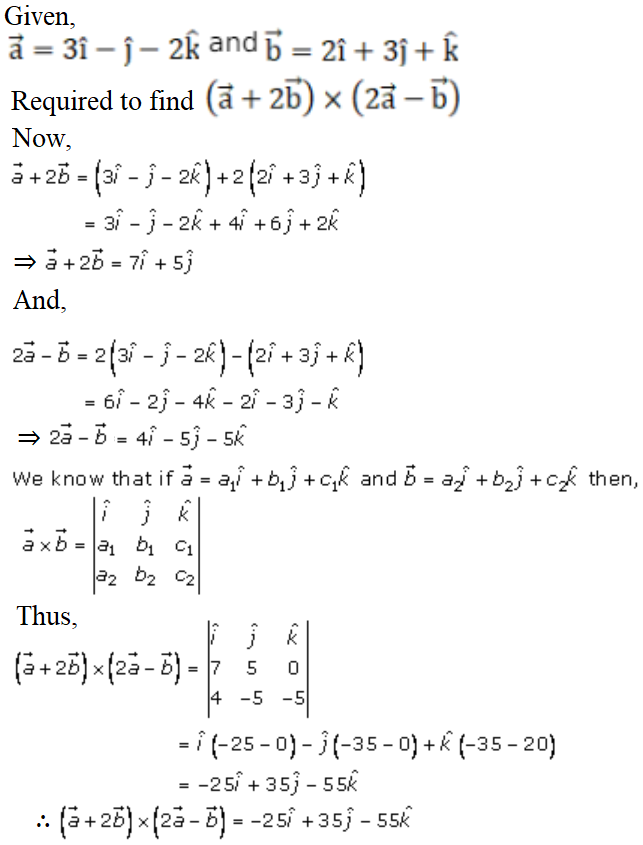# RD Sharma Solutions Class 12 Vector Or Cross Product Exercise 25.1

RD Sharma Solutions for Class 12 Maths Chapter 25 Vector or Cross Product Exercise 25.1 are enclosed here for students to access solutions at ease. These solutions are entirely as per the latest CBSE guidelines to help students score well in their board examination. For students practising these questions on a daily basis, achieving a high subject score in the final exam is ensured. Further, the RD Sharma Solutions for this chapter are given in an easily accessible PDF format, which can be downloaded from the link attached below.

## Download PDF of Rd Sharma Solution for Class 12 Maths Chapter 25 Exercise 1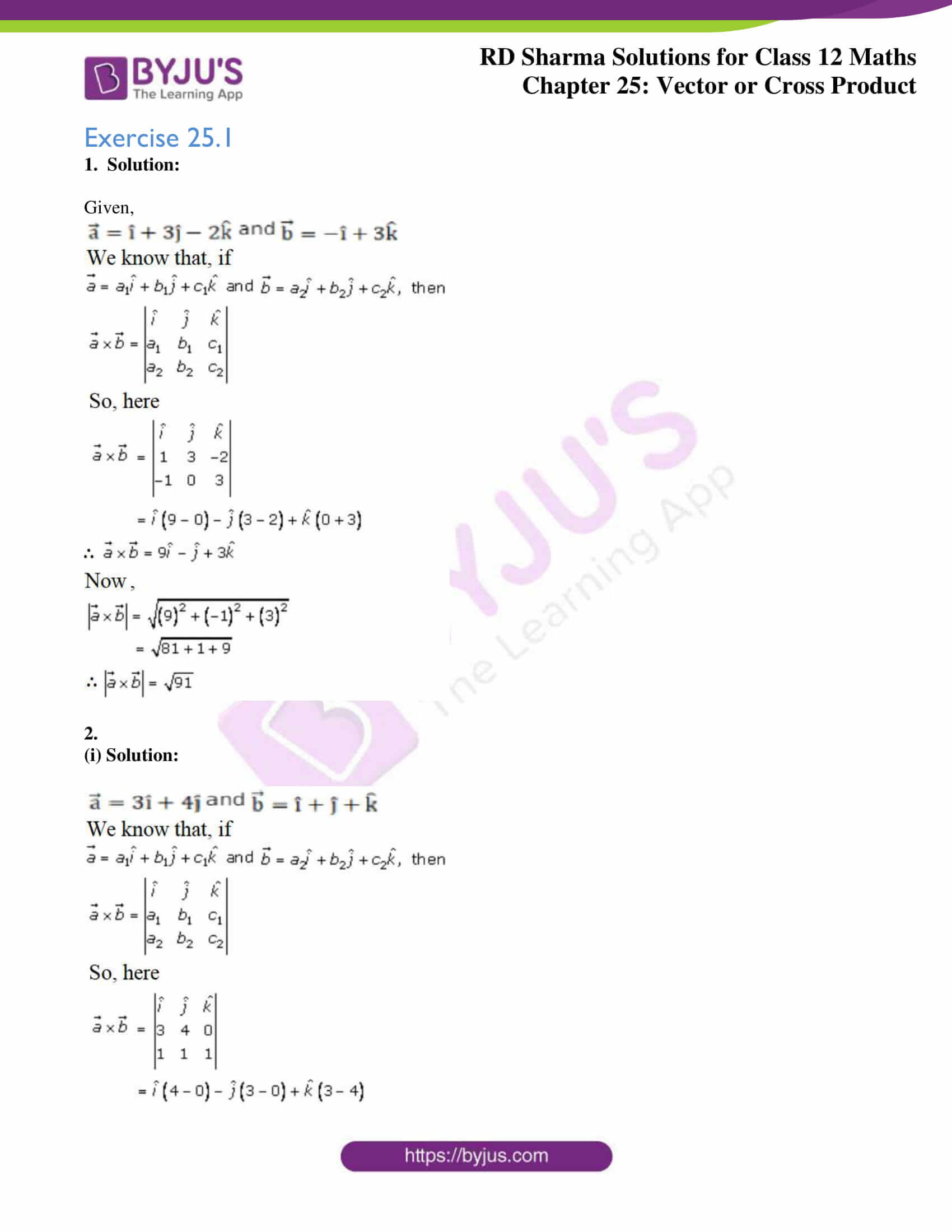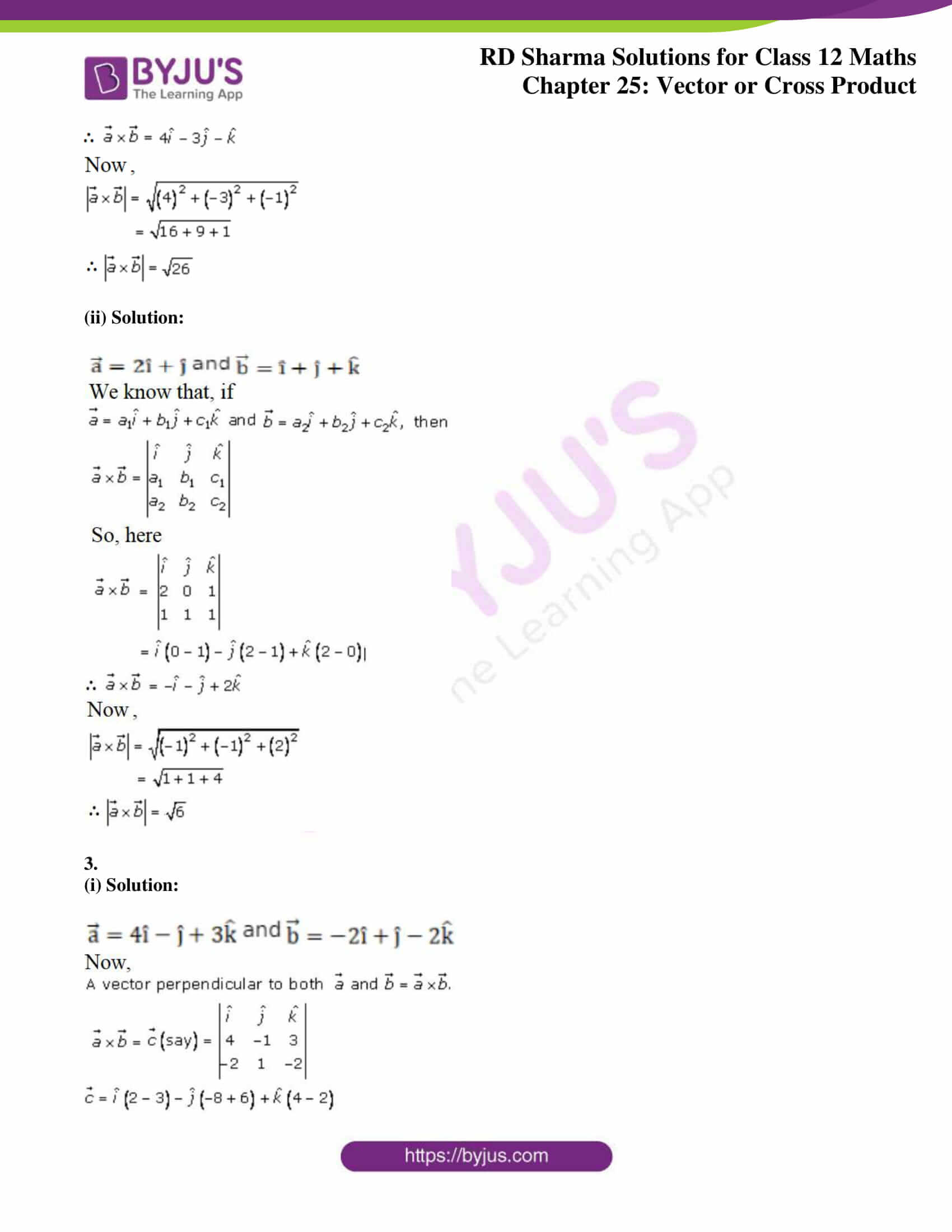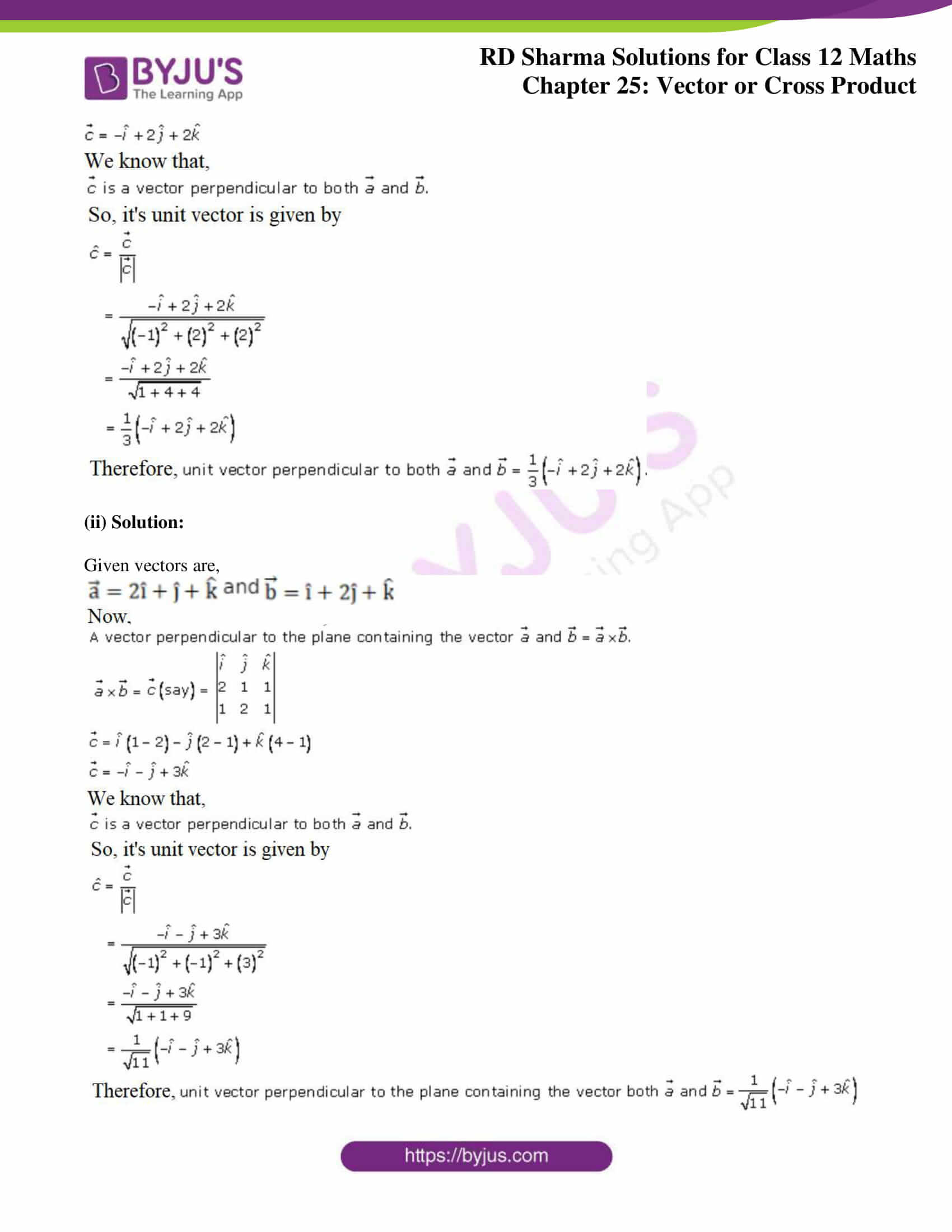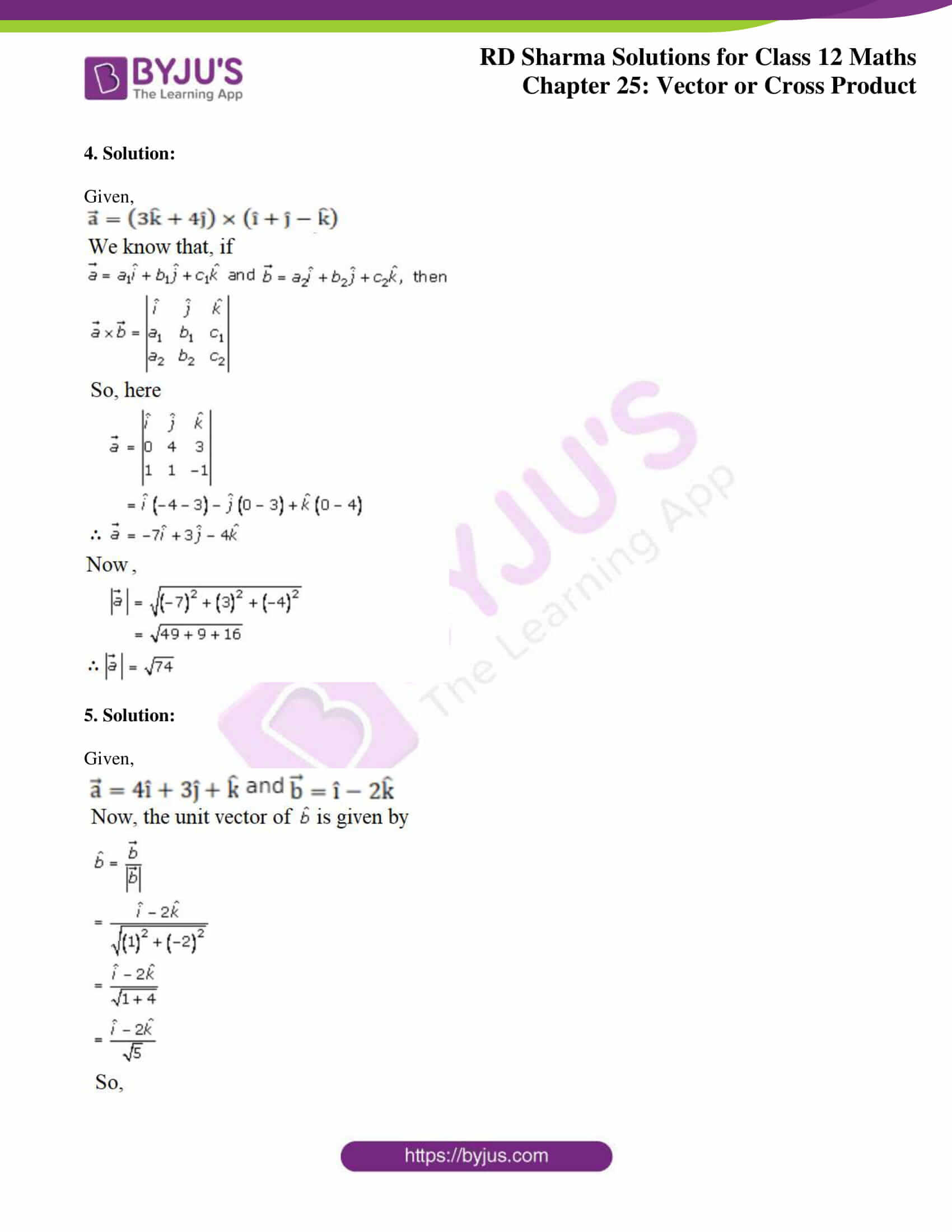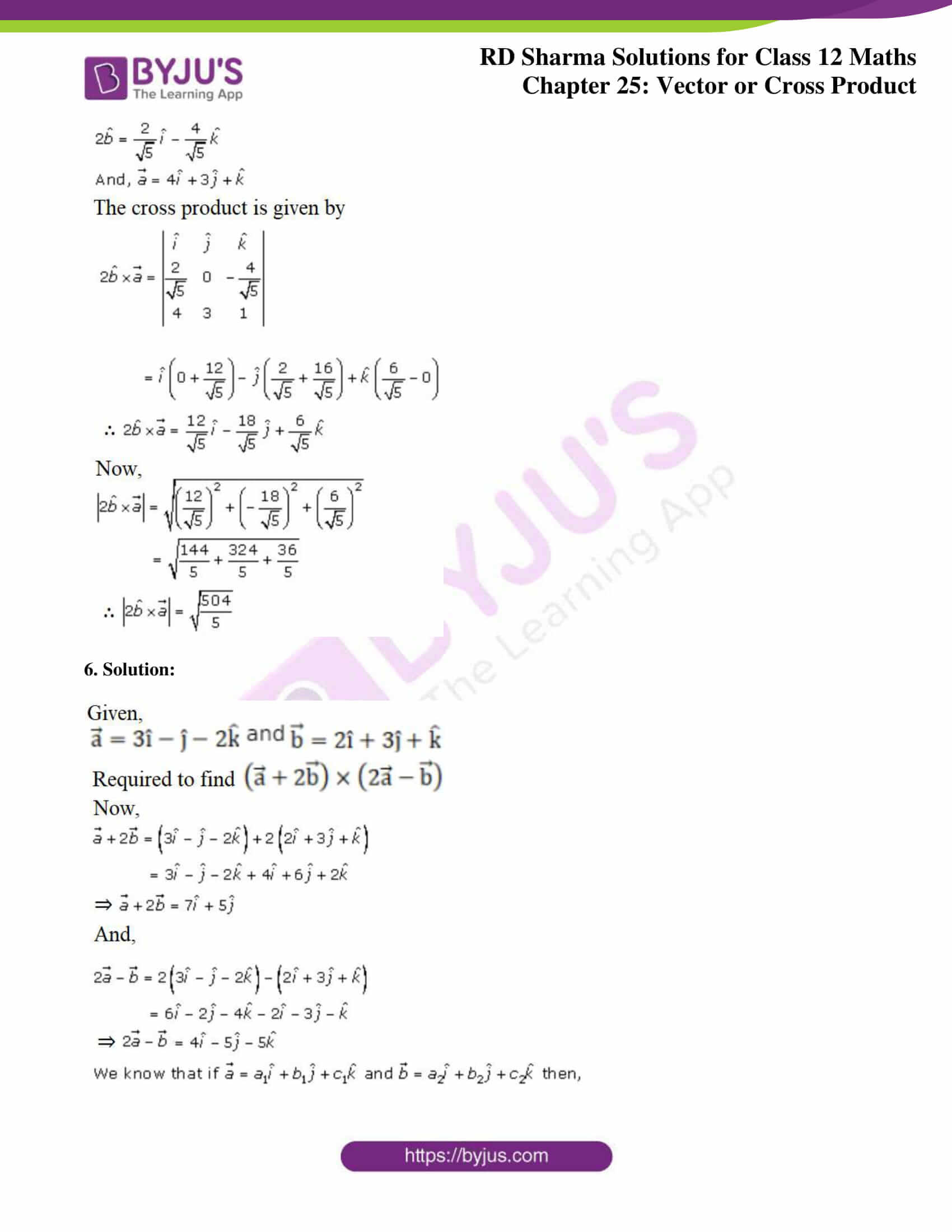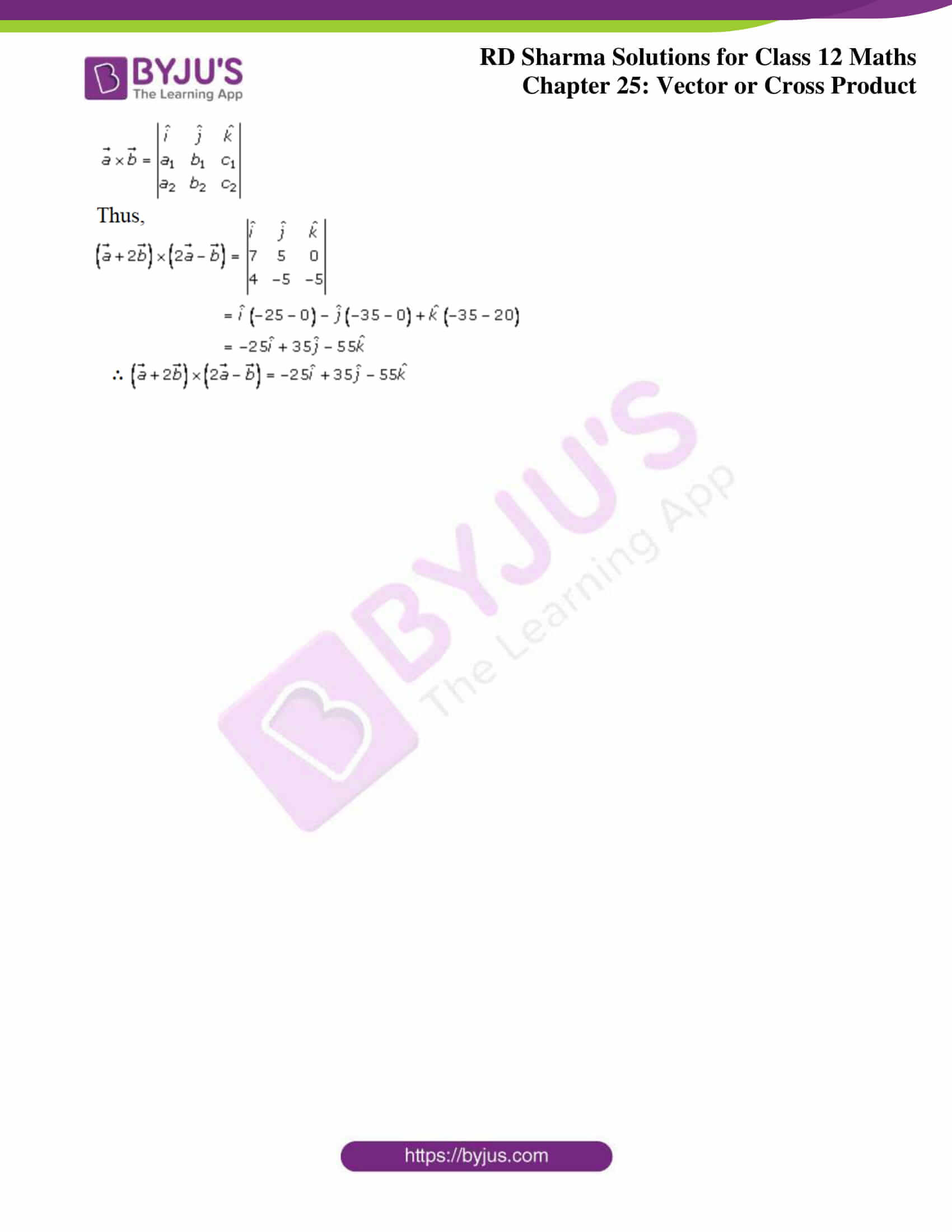### Access Answers for Rd Sharma Solution Class 12 Maths Chapter 25 Exercise 1

Exercise 25.1

1. Solution:

Given,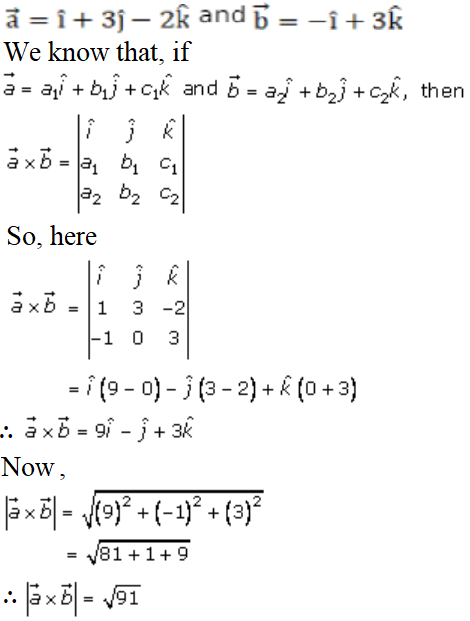2.

(i) Solution: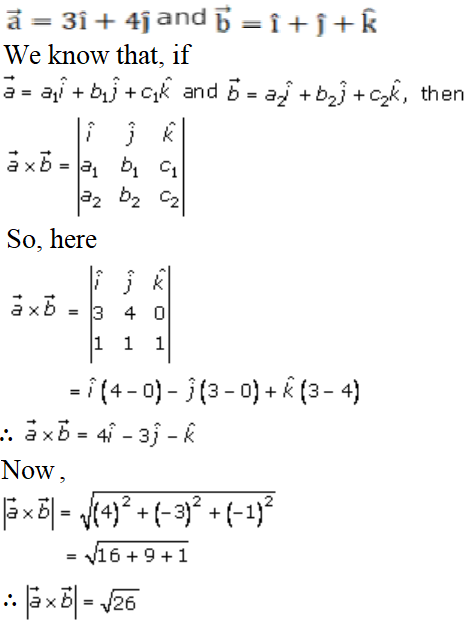(ii) Solution: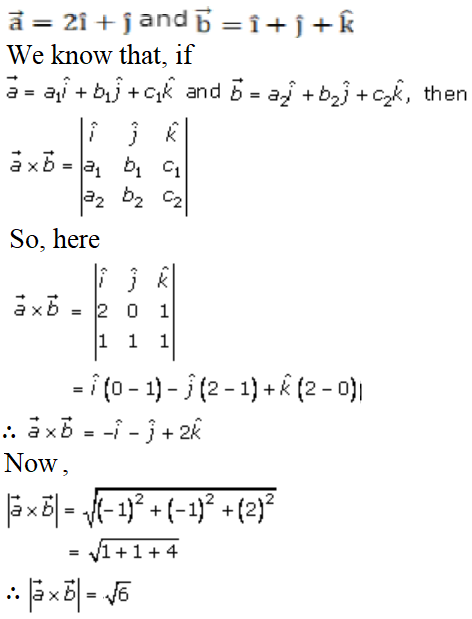3.

(i) Solution: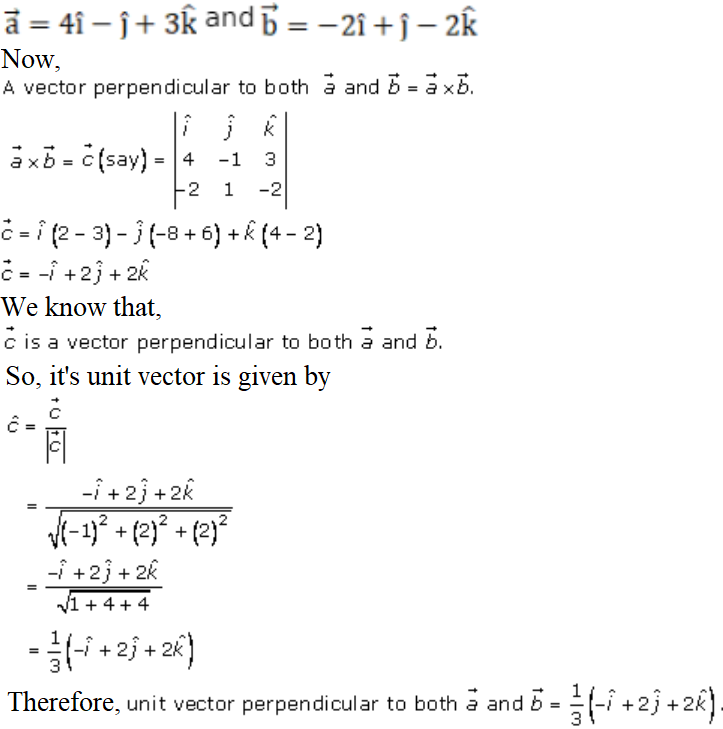(ii) Solution:

Given vectors are,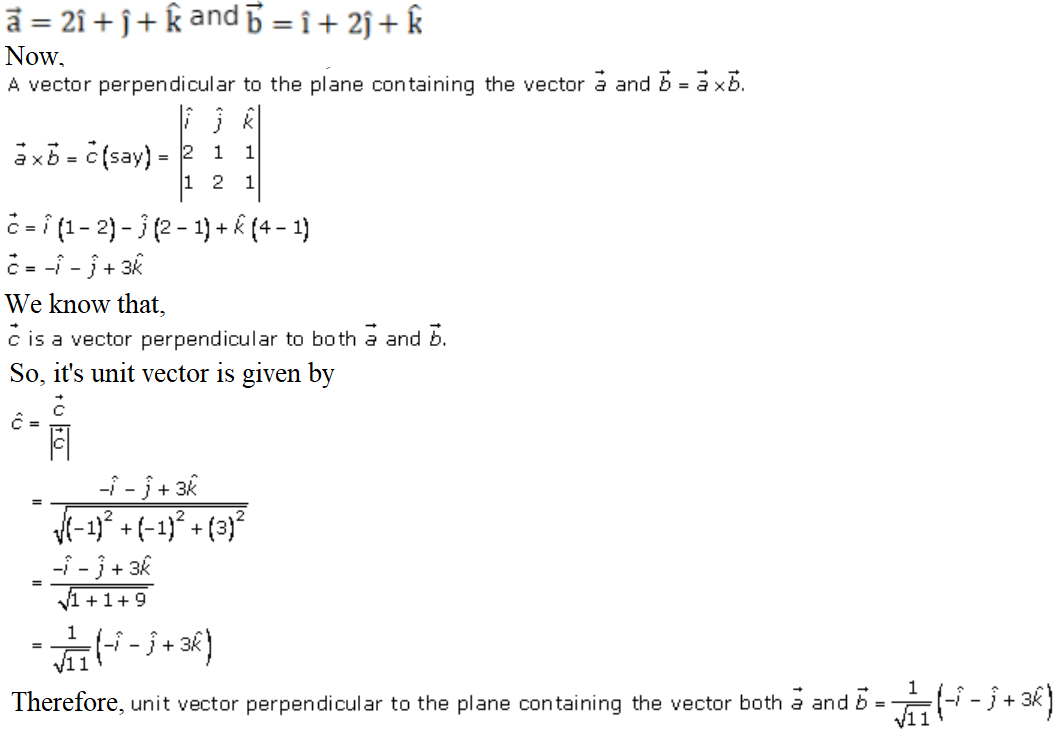4. Solution:

Given,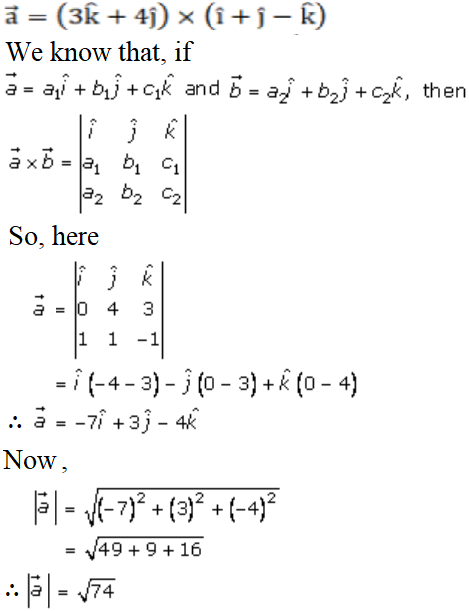5. Solution:

Given,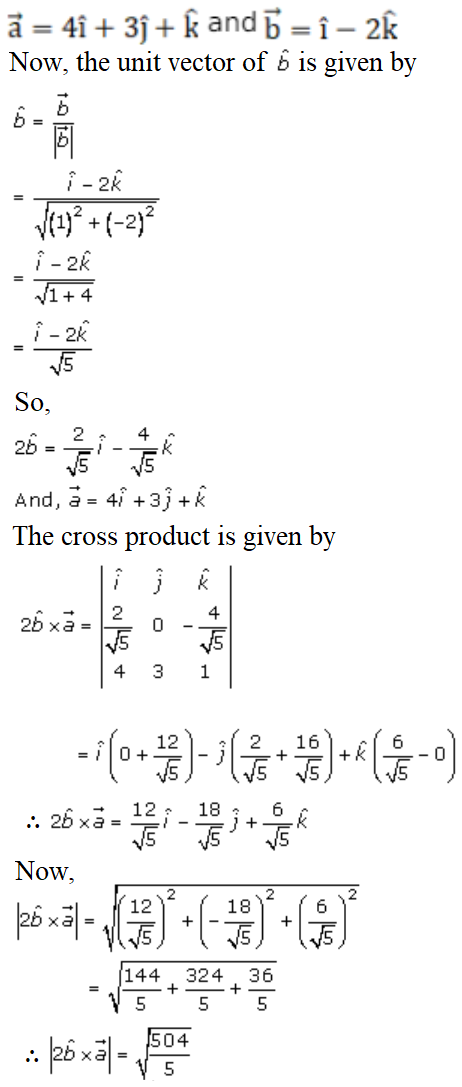6. Solution: